### Home > INT2 > Chapter 11 > Lesson 11.2.1 > Problem11-48

11-48.

Examine the diagrams below. For each one, use the geometric relationships to solve for the given variable.

1. $\overleftrightarrow { P R }$ is tangent to $⊙C$ at $P$ and
$m\overarc { P M O }= 314°$ . What is $QR$?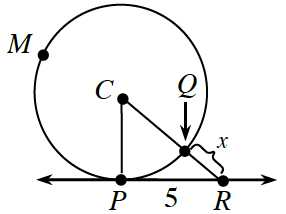1. radius $= 7$ units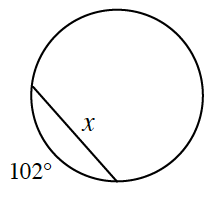Draw a diagram to help visualize the problem. Right triangle, C,P,R, horizontal leg, P R, labeled, 5, vertical leg, C P, labeled, r, angle, C, labeled theta. hypotenuse, C P, labeled, R, +, x. Point on side, C R, labeled, Q, & segment, Q R, labeled, x.

$θ = 360° - 314°$
$θ = 46°$Added to the triangle, angle c, labeled, 46 degrees.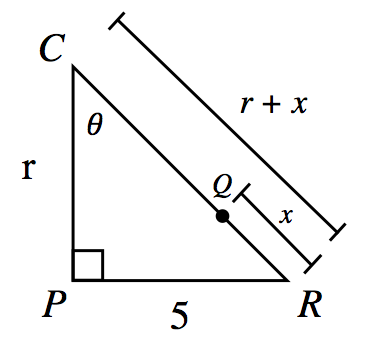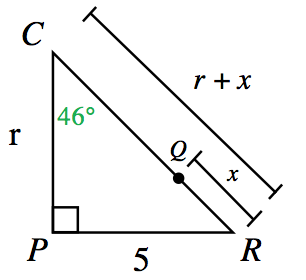Draw a diagram to help visualize the problem. Added to circle, segments from the center, to the end points of the chord, with dashed segment from center, perpendicular to the chord, creating 2 right triangles. Each radii labeled, 7 units, each central angle for the right angles, labeled theta.

$θ = 102° x 1/2$
$θ = 51°$Angles labeled theta, changed to label, 51 degrees.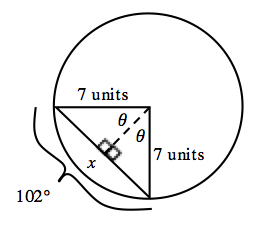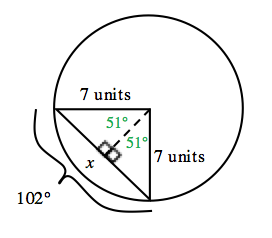$\text{tan}(46°)=\frac{5}{r}$

$r=\frac{5}{\text{tan}(46°)}$

$r ≈ 4.83$

$\text{sin}(46°)=\frac{5}{4.83+x}$

$4.83+x=\frac{5}{\text{sin}(46°)}$

$x ≈ 6.95 - 4.83$

$\text{sin}(51°)=\frac{\frac{x}{2}}{7}$

$\frac{x}{2}=7\text{sin}(51°)$

$x = 14\sin(51°)$

$QR ≈ 2.12$

$x ≈ 10.88$ units